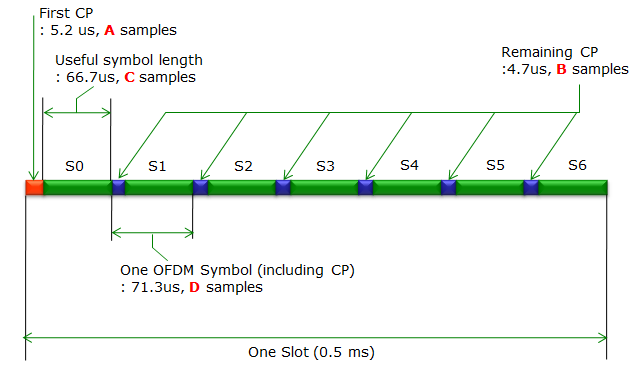LTE Quick Reference

Physical Layer Parameter - DL, FDD

Followings would be a quick cheatsheet if you are DSP engineer or FPGA engineer working in LTE PHY.

 Channel Bandwidth (Mhz) 1.4 3 5 10 15 20 Frame Duration (ms) 10 10 10 10 10 10 Sub carrier spacing (Khz) 15 15 15 15 15 15 Sampling Frequency (Mhz) 1.92 3.84 7.68 15.36 23.04 30.72 FFT Size 128 256 512 1024 1536 2048 Occupied Subcarriers (including DC) 73 181 301 601 901 1201 Guard Subcarriers 55 75 211 423 635 847 Number of Resource Blocks 6 15 25 50 75 100 Occupied Channel Bandwith (Mhz) 1.095 2.715 4.515 9.015 13.515 18.015 DL Bandwidth Efficiency 78.2% 90% 90% 90% 90% 90% OFDM Symbols for Subframe (for Short CP) 7 7 7 7 7 7 CP Length for Short CP (in us) 5.2 for the first symbol/4.69 for other symbols

Let's get into further details for some of the important parameters.

< Sampling Time >

• 20 Mhz BW Case :  1 sec / 30.72 Mhz = 1,000,000 us/30,720,000 Hz = 0.0326 us = 32.6 ns
• 15 Mhz BW Case :  1 sec / 23.04 Mhz = 1,000,000 us/23,040,000 Hz = 0.0434 us = 43.4 ns
• 10 Mhz BW Case :  1 sec / 15.36 Mhz = 1,000,000 us/15,360,000 Hz = 0.0652 us = 65.2 ns
• 5 Mhz BW Case :  1 sec / 7.68 Mhz = 1,000,000 us/7,680,000 Hz = 0.1302 us = 130.2 ns
• 3 Mhz BW Case :  1 sec / 3.84 Mhz = 1,000,000 us/3,840,000 Hz = 0.2604 us = 260.4 ns
• 1.4 Mhz BW Case :  1 sec / 1.92 Mhz = 1,000,000 us/1,920,000 Hz = 0.5208 us = 520.8 ns

< Number of Samples for each CP and OFDM Symbol >

Following illustration shows the number of samples in each CP and OFDM symbols for 20Mhz case. I will calculate the number of samples for other BW case by taking the ratio of FFT site of 20 Mhz and the FFT size of other BW.In short, I will list up the value of A,B,C,D for each system BW.Followings are A,B,C values for each BW

• 20 Mhz BW Case :
• A = 160
• B = 144
• C = 2048
• 15 Mhz BW Case :
• A = 160 x (FFT size for 15 Mhz/FFT size for 20 Mhz) = 160 x (1536/2048) = 120
• B = 144 x (FFT size for 15 Mhz/FFT size for 20 Mhz) = 144 x (1536/2048) = 108
• C = 2048 x (FFT size for 15 Mhz/FFT size for 20 Mhz) = 2048 x (1536/2048) = 1536
• 10 Mhz BW Case :
• A = 160 x (FFT size for 10 Mhz/FFT size for 20 Mhz) = 160 x (1024/2048) = 80
• B = 144 x (FFT size for 10 Mhz/FFT size for 20 Mhz) = 144 x (1024/2048) = 72
• C = 2048 x (FFT size for 10 Mhz/FFT size for 20 Mhz) = 2048 x (1024/2048) = 1024
• 5 Mhz BW Case :
• A = 160 x (FFT size for 5 Mhz/FFT size for 20 Mhz) = 160 x (512/2048) = 40
• B = 144 x (FFT size for 5 Mhz/FFT size for 20 Mhz) = 144 x (512/2048) = 36
• C = 2048 x (FFT size for 5 Mhz/FFT size for 20 Mhz) = 2048 x (512/2048) = 512
• 3 Mhz BW Case :
• A = 160 x (FFT size for 3 Mhz/FFT size for 20 Mhz) = 160 x (256/2048) = 20
• B = 144 x (FFT size for 3 Mhz/FFT size for 20 Mhz) = 144 x (256/2048) = 18
• C = 2048 x (FFT size for 3 Mhz/FFT size for 20 Mhz) = 2048 x (256/2048) = 256
• 1.4 Mhz BW Case :
• A = 160 x (FFT size for 1.4 Mhz/FFT size for 20 Mhz) = 160 x (128/2048) = 10
• B = 144 x (FFT size for 1.4 Mhz/FFT size for 20 Mhz) = 144 x (128/2048) = 9
• C = 2048 x (FFT size for 1.4 Mhz/FFT size for 20 Mhz) = 2048 x (128/2048) = 128

< BandWidth/Guardband Width >• 20 Mhz BW Case :
• A = Occupied Subcarriers (including DC)  x 15 Khz = 1201 x 15 Khz = 18015 Khz = 18.015 Mhz
• B = Occupied Subcarriers (including DC) = 1201
• C + D = Guard Subcarriers = 847
• 15 Mhz BW Case :
• A = Occupied Subcarriers (including DC)  x 15 Khz = 901 x 15 Khz = 13515 Khz = 13.515 Mhz
• B = Occupied Subcarriers (including DC) = 901
• C + D = Guard Subcarriers = 635
• 10 Mhz BW Case :
• A = Occupied Subcarriers (including DC)  x 15 Khz = 601 x 15 Khz = 9015 Khz = 9.015 Mhz
• B = Occupied Subcarriers (including DC) = 601
• C + D = Guard Subcarriers = 423
• 5 Mhz BW Case :
• A = Occupied Subcarriers (including DC)  x 15 Khz = 301 x 15 Khz = 4515 Khz = 4.515 Mhz
• B = Occupied Subcarriers (including DC) = 301
• C + D = Guard Subcarriers = 211
• 3 Mhz BW Case :
• A = Occupied Subcarriers (including DC)  x 15 Khz = 151 x 15 Khz = 2265 Khz = 2.265 Mhz
• B = Occupied Subcarriers (including DC) = 151
• C + D = Guard Subcarriers = 105
• 1.4 Mhz BW Case :
• A = Occupied Subcarriers (including DC)  x 15 Khz = 76 x 15 Khz = 1140 Khz = 1.140 Mhz
• B = Occupied Subcarriers (including DC) = 76
• C + D = Guard Subcarriers = 52